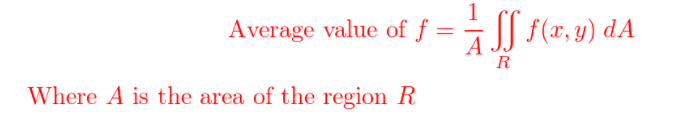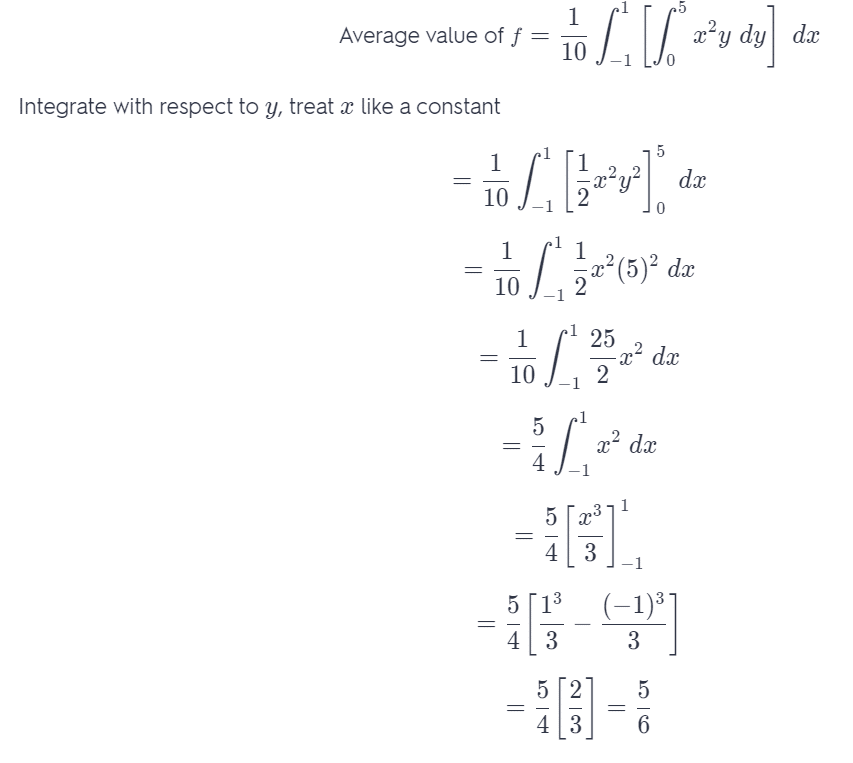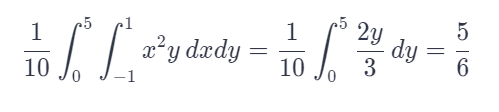# Find The Average Value Of F Over The Given Rectangle.

We thoroughly check each answer to a question to provide you with the most correct answers. Found a mistake? Let us know about it through the REPORT button at the bottom of the page.

Find the average value of f over the given rectangle.
f (x, y) = x^2y,
R has vertices (-1, 0), (-1, 5), (1, 5), (1, 0)

## Explanation

Recall that the average value of the integral is obtained by dividing the integral of the function with the area we are integrating over. Since our area is a rectangle given with the segments: [-1, 1] x [0, 5] the area of the rectangle is equal to 2 * 5 = 10.We calculate the integral: